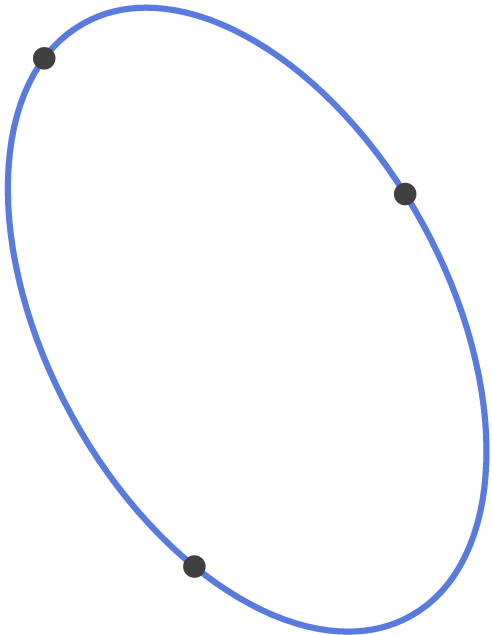# Is it a Circle?An ellipse passes through the points $(0,-1)$, $(2,0)$, and $(-2,2)$ and encloses minimum possible area.

$Ax^2+Bxy+Cy^2+Dx+Ey+F=0$ is the equation representing the ellipse, where $A$ and $B$ are positive coprime integers.

Find $A+B+C+D+E+F$.

×